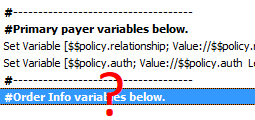Select Page
• What: Dynamically Add and Retrieve More Data to and from a Single Variable (A “Digging Deep” article)
• With: All Versions
• Why: You want to set a variable, but it’s possible that there may be more than one instance of that variable.Description of the problem:
I ran into a problem today while parsing a page of patient information where I needed to set a variable for an item on that page.  However, I discovered that sometimes that item occurred more than once on the page but with a new value (for example: this might be the case with multiple phone numbers or emails.  In my case, it was order numbers since sometimes the doctor would order more than one procedure for a patient).  On top of that, sometimes the order info appeared on a second page as overflow from the first page.

My first instinct (my beginner instinct) was to just set a few variables to look for more instances.  For example, I was going to name the variable \$\$order (it is a global variable because I need to hold on to it beyond the scope of the script it lives in).  Then make a couple more like this: \$\$order2, \$\$order3, etc.  The problem was that I started discovering that some cases showed up to 5 of them…and who knows if a strange outlier would come in with even more?!

Here’s my solution:
I realized that if I kept adding to the same variable \$\$order, as long as each new order was on a new line in the variable, I could then retrieve each order using the GetValue function.

Let’s talk about how the GetValue function works.

• The GetValue function requires two parameters seen here: GetValue ( listOfValues ; valueNumber ).
• The “listOfValues” in my case is the \$\$order variable.
• The “valueNumber” corresponds to the “line number” that each value is on.  For example, lets say I have 3 orders.  As I collect each one, I apply them to the \$\$order variables with the paragraph return (¶) separating each one.  It might end up looking like this:
order1¶
order2¶
order3¶
In this case, setting the “valueNumber” to 3 would give me the value “order3” because it is on line 3.
• To populate your variable with more lines, you simply take the original variable and set it to itself then concatenate the new info with a trailing ¶.  For example, if I set \$\$order = “order1¶”, then when I need to add the next order I simply set it like this: \$\$order = \$\$order & “order2¶”.  So far this would set the \$\$order variable to:
order1¶
order2¶
• To get the number of items in your variable, you can use the ValueCount ( text ) function.  Simply set the \$\$order variable as the parameter like this ValueCount ( \$\$order ) and it will return the number of lines in your variable.
• Finally, to pull out a line of data from your variable we can go back to the GetValue ( listOfValues ; valueNumber ) function and pull each line using a loop.  That might look something like this:
# \$valueCount gives me the number of lines in my variable
Set Variable [\$valueCount, Value: ValueCount ( \$\$order ) ]
# \$lineCount is a “counter” variable that will increment until it equals the \$valueCount, then exits the loop
# \$lineCount can also be used as the valueNumber in GetValue ( listOfValues ; valueNumber )
Set Variable [ \$lineCount; Value: 1 ] //we start with 1 then increment
Loop
Set Field [ table::order; GetValue (\$\$order; \$lineCount)]
Set Variable [\$lineCount; Value: \$lineCount +1] //here we add 1 to \$lineCount itself
Exit Loop If [\$lineCount = \$valueCount]
New Record/Request
End Loop

Bottom line:
Ultimately, the focus of this article was to be able to add more data to the same variable, then take advantage of the GetValue function to pull each piece of data out individually.